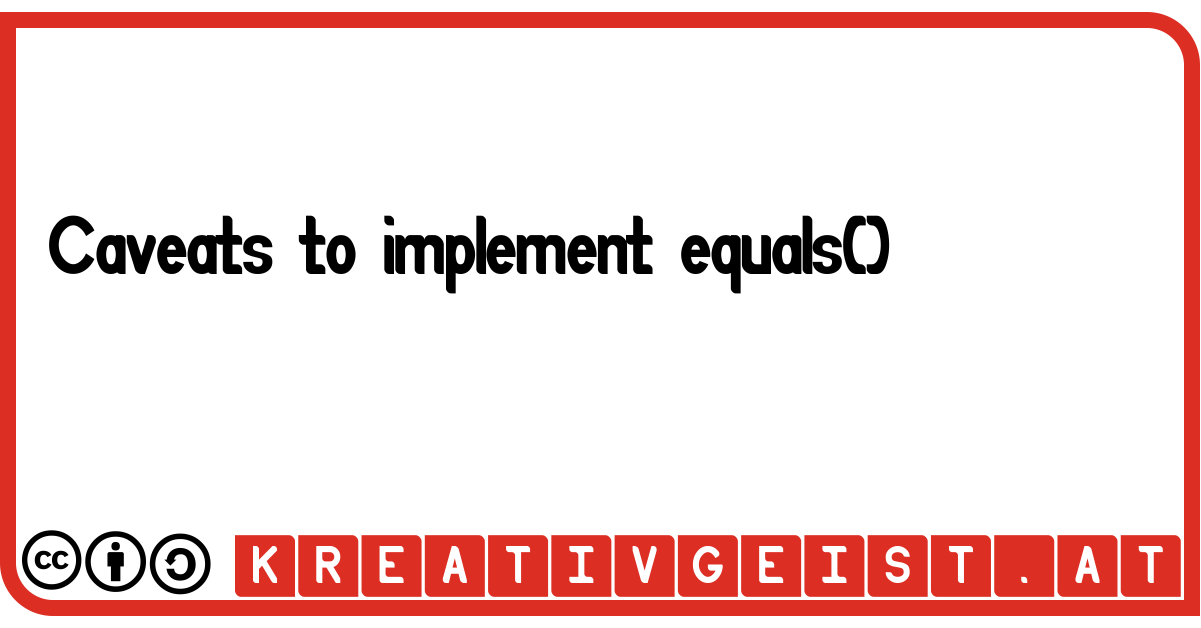# Equals - A quick implementation

If you need to compare 2 objects, you will need to implement the equals()-function.
But what is a short implementation of all needed cases?

## The problem

In Java `equals()` is a built-in function of Object to compare it with another object. Since every object inherits this function, it must be overridden to ensure it works as expected.
The standard-implementation only compares if the references are equal, because there are no more attributes of object, that could be compared for equality.

## The Implementation

First of all, it is important to use the exact signature. So it is necessary to pass Object as parameter, not the type of the class you are implementing. Otherwise it could be possible that the standard-implementation of equals() could be called in some unexpected cases.
Here it is highly recommended to use the @Override directive to prevent a possible mistake in the signature when implementing it.
```@Override
public boolean equals(Object o) {
}```
Since there is the possibility to pass a non-matching object to the equals-function, the type of the argument must be taken into account. If the argument is null, the result should always be false. Also any unexpected type as argument must return false.
Therefore the getClass()-function or instanceof can be used. The second one has the benefit to handle a null-parameter implicitly, so in that case you can omit the mandatory check for null. After checking that, a casting to the desired class is possible safely.
```if (o != null && getClass() == o.getClass()) {
MyObject that = (MyObject) o;
}
// or
if (o instanceof MyObject) {
MyObject that = (MyObject) o;
}
```
Now you can compare all desired members to determine, if the objects are equal. As a nice benefit, you don't need getters for all checked variables, since the private-keyword defines access at class-level not object-level. So an object can access all private variables of an object of the same type.
For speeding up the comparation of an object to itself, the reference can be compared.
```if (o == this) {
result = true;
}
```

## Full example

A full example of an equals-implementation could look like this:
```public boolean equals(Object o) {
boolean result = false;

if (o == this) {
result = true;
}
else if (o instanceof MyObject) {
MyObject that = (MyObject) o;
result = (this.a == that.a);
}
return result;
}
```

## Accordance with hashCode

Keep in mind that the Java 9 definition of hashCode needs to be in accordance with equals. Particularly if two objects are equal (by means of equals) then both of them must return the same value for hashCode. That means every attribute used to calculate the hashCode must also be taken into account for the equals-comparation!

## Conclusion

To implement a fully functional equals-function, you should:
1. Override the exact signature of equals of object and pass an Object as parameter
2. Check the passed parameter for null
3. Check the passed parameter for the correct type and cast it
4. Compare the values of all desired attributes (without using getters)
5. Use all attributes used to calculate the value hashCode() also for comparison in equals
6. You can also compare two objects to be equal by comparing their reference